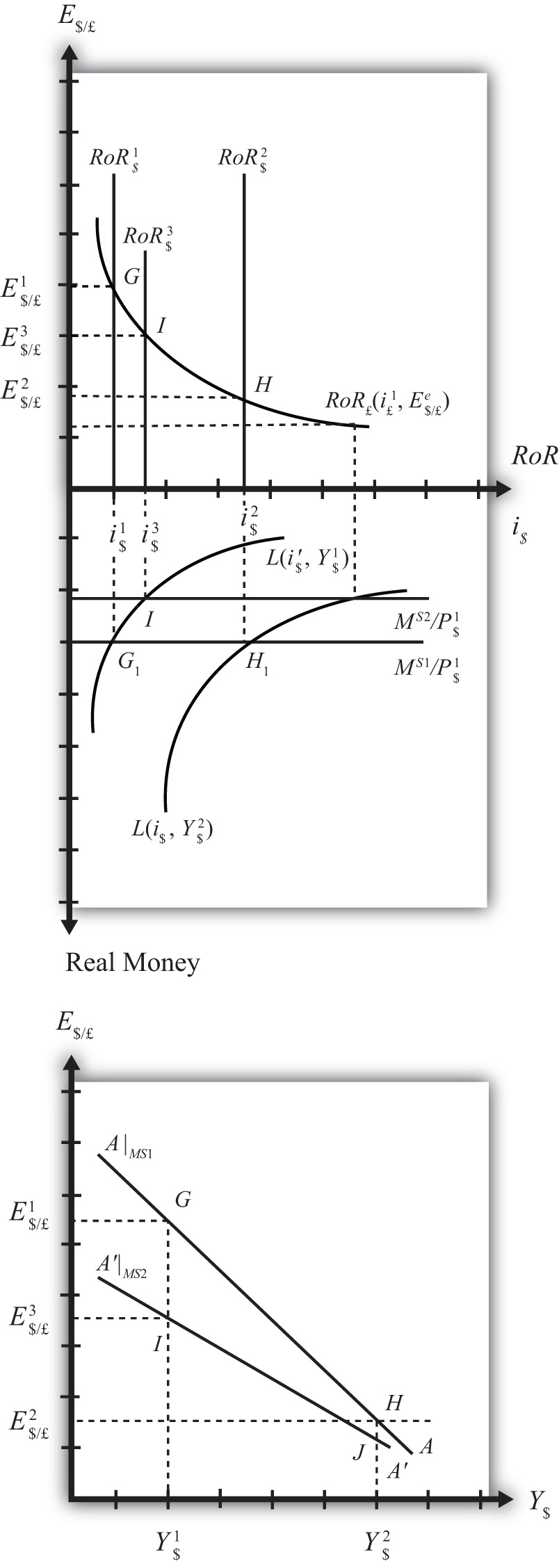9.5 Shifting the AA Curve

Learning Objective

1. Learn which exogenous variables will shift the AA curve and in which direction.

The AA curve depicts the relationship between changes in one exogenous variable and one endogenous variable within the asset market model. The exogenous variable changed is gross national product (GNP). The endogenous variable affected is the exchange rate. At all points along the AA curve, it is assumed that all other exogenous variables remain fixed at their original values.

The AA curve will shift if there is a change in any of the other exogenous variables. We illustrate how this works in Figure 9.6 "AA Curve Effects from a Decrease in the Money Supply", where we assume that the money supply in the economy falls from its initial level MS1 to a lower level MS2.

Figure 9.6 AA Curve Effects from a Decrease in the Money SupplyAt the initial money supply (MS1) and initial GNP level Y\$1, real money demand intersects real money supply at point G, determining the interest rate i\$1. This in turn determines the rate of return on U.S. assets, RoR\$1, which intersects the foreign British RoR£ at G in the upper diagram, determining the equilibrium exchange rate E\$/£1. If the money supply and all other exogenous variables remain fixed, while GNP increases to Y\$2, the equilibriums shift to points H in the lower and upper diagrams, determining exchange rate E\$/£2. This exercise plots out the initial AA curve labeled AA|MS1 in the lower diagram connecting points G and H. Note, AA|MS1 is read as “the AA curve given that MS = MS1.”

Now, suppose the money supply MS falls to MS2. The reduction in MS leads to a reduction in the real money supply, which, at GNP level Y\$1, shifts the money market equilibrium to point I, determining a new interest rate, i\$3. In the Forex market, the rate of return rises to RoR\$3, which determines the equilibrium exchange rate E\$/£3. The equilibriums at points I corresponding to the combination (Y\$1, E\$/£3) are transferred to point I on the lower diagram. This point lies on a new AA curve because a second exogenous variable, namely, MS, has changed. If we maintain the money supply at MS2 and change the GNP up to Y\$2, the equilibrium will shift to point J (shown only on the lower diagram), plotting out a whole new AA curve. This AA curve is labeled AA′|MS2, which means “the AA curve given that MS = MS2.”

The effect of a decrease in the money supply is to shift the AA curve downward. Indeed, a change in any exogenous variable in the asset markets that reduces the equilibrium exchange rate, except a change in GNP, will cause the AA curve to shift down. Likewise, any change in an exogenous variable that causes an increase in the exchange rate will cause the AA curve to shift up. A change in GNP will not shift AA because its effect is accounted for by the AA curve itself. Note that curves or lines can shift only when a variable not plotted on the axis changes.

The following table presents a list of all variables that can shift the AA curve up and down. The up arrow indicates an increase in the variable, and a down arrow indicates a decrease.

 AA up-shifters ↑MS ↓P\$ ↑i£ ↑E\$/£e AA down-shifters ↓MS ↑P\$ ↓i£ ↓E\$/£e

Refer to Chapter 5 "Interest Rate Parity" and Chapter 7 "Interest Rate Determination" for a complete description of how and why each variable affects the exchange rate. For easy reference though, recall that MS is the U.S. money supply, P\$ is the U.S. price level, i£ is the foreign British interest rate, and E\$/£e is the expected future exchange rate.

Key Takeaways

• The effect of an increase in the money supply (or a decrease in the price level, an increase in foreign interest rates, or an increase in the expected exchange rate [as defined]) is to shift the AA curve upward.
• The effect of a decrease in the money supply (or an increase in the price level, a decrease in foreign interest rates, or a decrease in the expected exchange rate [as defined]) is to shift the AA curve downward.

Exercise

1. Identify whether the AA curve shifts in response to each of the following changes. Indicate whether the curve shifts up, down, left, or right. Possible answers are AA right, AA left, or neither.

1. Decrease in government transfer payments.
2. Decrease in the foreign price level.
3. Increase in foreign interest rates.
4. Decrease in the expected exchange rate E\$/£e.
5. Decrease in U.S. GNP.
6. Decrease in the U.S. money supply.